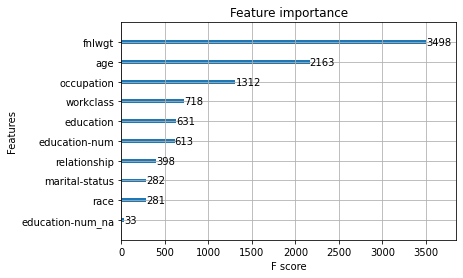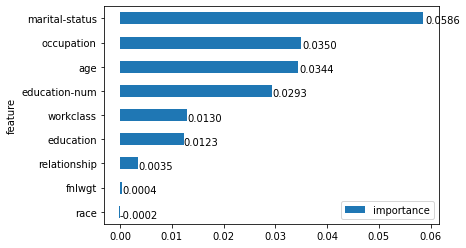## Lesson Video:

This article is also a Jupyter Notebook available to be run from the top down. There will be code snippets that you can then run in any environment.

Below are the versions of fastai, fastcore, wwf, xgboost, sklearn, and rfpimp currently running at the time of writing this:

• fastai: 2.1.10
• fastcore: 1.3.13
• wwf: 0.0.8
• xgboost: 0.90
• sklearn: 0.0
• rfpimp: 1.3.6

Often for tabular problems, we deal with ensembling from other models. For today, we'll look at using XGBoost (Gradient Boosting) mixed in with fastai, and you'll notice we'll be using fastai to prepare our data!

from fastai.tabular.all import *


Let's first build our TabularPandas object:

path = untar_data(URLs.ADULT_SAMPLE)

cat_names = ['workclass', 'education', 'marital-status', 'occupation', 'relationship', 'race']
cont_names = ['age', 'fnlwgt', 'education-num']
procs = [Categorify, FillMissing, Normalize]
y_names = 'salary'
y_block = CategoryBlock()
splits = RandomSplitter()(range_of(df))

to = TabularPandas(df, procs=procs, cat_names=cat_names, cont_names=cont_names,
y_names=y_names, y_block=y_block, splits=splits)


## XGBoost

import xgboost as xgb


We'll need our x's and our y's

X_train, y_train = to.train.xs, to.train.ys.values.ravel()
X_test, y_test = to.valid.xs, to.valid.ys.values.ravel()

model = xgb.XGBClassifier(n_estimators = 100, max_depth=8, learning_rate=0.1, subsample=0.5)


And now we can fit our classifier:

xgb_model = model.fit(X_train, y_train)


And we'll grab the raw probabilities from our test data:

xgb_preds = xgb_model.predict_proba(X_test)

xgb_preds

array([[0.89155704, 0.10844298],
[0.6882768 , 0.31172317],
[0.79331285, 0.20668715],
...,
[0.49610275, 0.50389725],
[0.90957344, 0.09042657],
[0.9879613 , 0.01203871]], dtype=float32)

And check it's accuracy

accuracy(tensor(xgb_preds), tensor(y_test))

tensor(0.8340)

We can even plot the importance

from xgboost import plot_importance

plot_importance(xgb_model)

<matplotlib.axes._subplots.AxesSubplot at 0x7f16d079ca20>## Bring in fastai

dls = to.dataloaders()

learn = tabular_learner(dls, layers=[200,100], metrics=accuracy)

learn.fit(5, 1e-2)

epoch train_loss valid_loss accuracy time
0 0.378514 0.372543 0.829545 00:04
1 0.363559 0.371811 0.827242 00:04
2 0.356018 0.362737 0.830313 00:04
3 0.359589 0.359365 0.836609 00:04
4 0.343187 0.362838 0.838452 00:04

As we can see, our neural network has 83.84%, slighlty higher than the GBT

Now we'll grab predictions

nn_preds = learn.get_preds()

nn_preds

tensor([[0.9685, 0.0315],
[0.6587, 0.3413],
[0.5426, 0.4574],
...,
[0.3229, 0.6771],
[0.9517, 0.0483],
[0.9978, 0.0022]])

Let's check to see if our feature importance changed at all

class PermutationImportance():
"Calculate and plot the permutation importance"
def __init__(self, learn:Learner, df=None, bs=None):
"Initialize with a test dataframe, a learner, and a metric"
self.learn = learn
self.df = df if df is not None else None
bs = bs if bs is not None else learn.dls.bs
self.dl = learn.dls.test_dl(self.df, bs=bs) if self.df is not None else learn.dls
self.x_names = learn.dls.x_names.filter(lambda x: '_na' not in x)
self.na = learn.dls.x_names.filter(lambda x: '_na' in x)
self.y = dls.y_names
self.results = self.calc_feat_importance()
self.plot_importance(self.ord_dic_to_df(self.results))

def measure_col(self, name:str):
"Measures change after column shuffle"
col = [name]
if f'{name}_na' in self.na: col.append(name)
orig = self.dl.items[col].values
perm = np.random.permutation(len(orig))
self.dl.items[col] = self.dl.items[col].values[perm]
metric = learn.validate(dl=self.dl)
self.dl.items[col] = orig
return metric

def calc_feat_importance(self):
"Calculates permutation importance by shuffling a column on a percentage scale"
print('Getting base error')
base_error = self.learn.validate(dl=self.dl)
self.importance = {}
pbar = progress_bar(self.x_names)
print('Calculating Permutation Importance')
for col in pbar:
self.importance[col] = self.measure_col(col)
for key, value in self.importance.items():
self.importance[key] = (base_error-value)/base_error #this can be adjusted
return OrderedDict(sorted(self.importance.items(), key=lambda kv: kv, reverse=True))

def ord_dic_to_df(self, dict:OrderedDict):
return pd.DataFrame([[k, v] for k, v in dict.items()], columns=['feature', 'importance'])

def plot_importance(self, df:pd.DataFrame, limit=20, asc=False, **kwargs):
"Plot importance with an optional limit to how many variables shown"
df_copy = df.copy()
df_copy['feature'] = df_copy['feature'].str.slice(0,25)
df_copy = df_copy.sort_values(by='importance', ascending=asc)[:limit].sort_values(by='importance', ascending=not(asc))
ax = df_copy.plot.barh(x='feature', y='importance', sort_columns=True, **kwargs)
for p in ax.patches:
ax.annotate(f'{p.get_width():.4f}', ((p.get_width() * 1.005), p.get_y()  * 1.005))

imp = PermutationImportance(learn)

Getting base error

Calculating Permutation Importance

100.00% [9/9 00:05<00:00]And it did! Is that bad? No, it's actually what we want. If they utilized the same things, we'd expect very similar results. We're bringing in other models to hope that they can provide a different outlook to how they're utilizing the features (hopefully differently)

## Ensembling

And perform our ensembling! To do so we'll average our predictions to gather (take the sum and divide by 2)

avgs = (nn_preds + xgb_preds) / 2

avgs

tensor([[0.9300, 0.0700],
[0.6735, 0.3265],
[0.6679, 0.3321],
...,
[0.4095, 0.5905],
[0.9307, 0.0693],
[0.9929, 0.0071]])

And now we'll take the argmax to get our predictions:

argmax = avgs.argmax(dim=1)

argmax

tensor([0, 0, 0,  ..., 1, 0, 0])

How do we know if it worked? Let's grade our predictions:

y_test

array([0, 0, 0, ..., 0, 1, 0], dtype=int8)
accuracy(tensor(nn_preds), tensor(y_test))

tensor(0.8385)
accuracy(tensor(xgb_preds), tensor(y_test))

tensor(0.8340)
accuracy(tensor(avgs), tensor(y_test))

tensor(0.8391)

As you can see we scored a bit higher!

## Bringing in Random Forests

Let's also try with Random Forests

from sklearn.ensemble import RandomForestClassifier

tree = RandomForestClassifier(n_estimators=100)


Now let's fit

tree.fit(X_train, y_train);


Now, we are not going to use the default importances. Why? Read up here:

Beware Default Random Forest Importances by Terence Parr, Kerem Turgutlu, Christopher Csiszar, and Jeremy Howard

Instead, based on their recommendations we'll be utilizing their rfpimp package

from rfpimp import *

imp = importances(tree, X_test, to.valid.ys)

plot_importances(imp)


Which as we can see, was also very different.

Now we can get our raw probabilities:

forest_preds = tree.predict_proba(X_test)

forest_preds

array([[0.99, 0.01],
[0.72, 0.28],
[0.75, 0.25],
...,
[0.42, 0.58],
[0.72, 0.28],
[1.  , 0.  ]])

And now we can add it to our ensemble:

avgs = (nn_preds + xgb_preds + forest_preds) / 3

accuracy(tensor(avgs), tensor(y_test))

tensor(0.8354)

As we can see, it didn't quite work how we wanted to. But that is okay, the goal was to experiment!